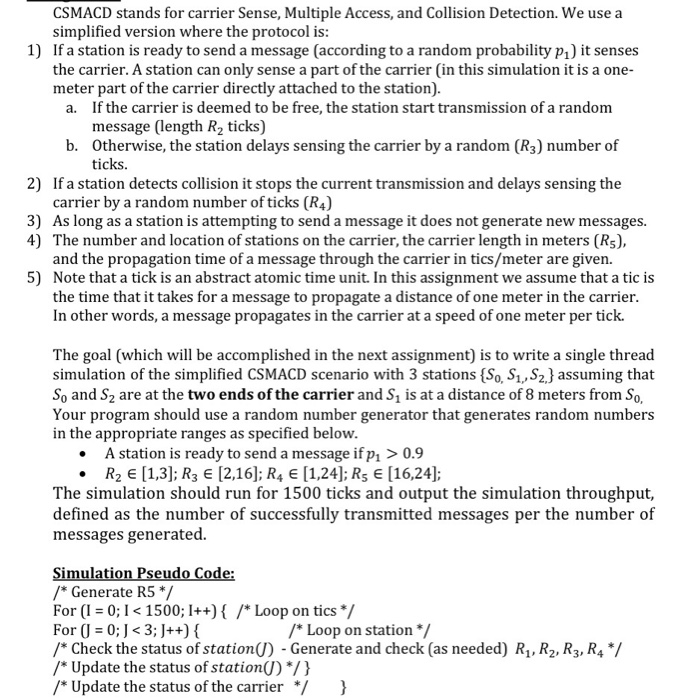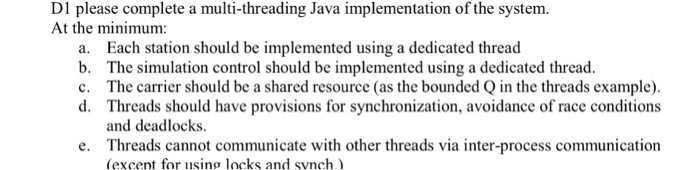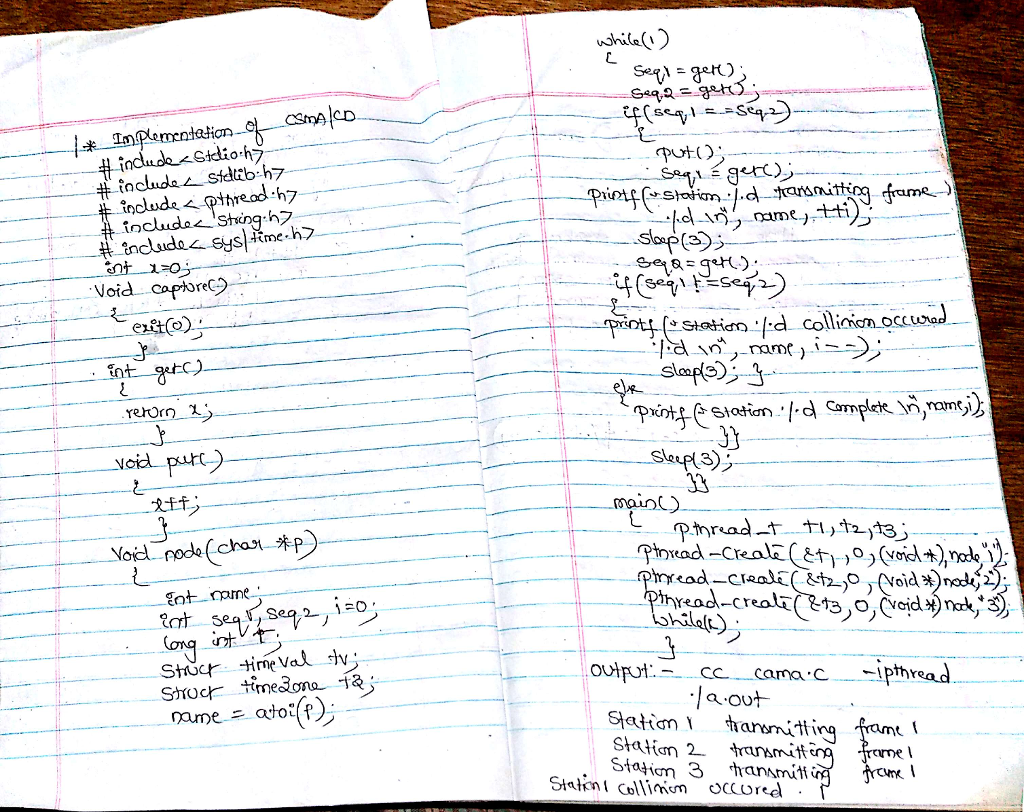# Question & Answer: CSMACD stands for carrier Sense, Multiple Access, and Collision Detection. We use a simplified version where the protocol i:s 1) If a station i…..CSMACD stands for carrier Sense, Multiple Access, and Collision Detection. We use a simplified version where the protocol i:s 1) If a station is ready to send a message (according to a random probability pi) it senses the carrier. A station can only sense a part of the carrier (in this simulation it is a one- meter part of the carrier directly attached to the station). If the carrier is deemed to be free, the station start transmission of a random message (length R2 ticks) a. Otherwise, the station delays sensing the carrier by a random (R3) number of ticks. b. 2) If a station detects collision it stops the current transmission and delays sensing the carrier by a random number of ticks (R4) As long as a station is attempting to send a message it does not generate new messages. The number and location of stations on the carrier, the carrier length in meters (Rs), and the propagation time of a message through the carrier in tics/meter are given. Note that a tick is an abstract atomic time unit. In this assignment we assume that a tic is the time that it takes for a message to propagate a distance of one meter in the carrier In other words, a message propagates in the carrier at a speed of one meter per tick. 3) 4) 5) The goal (which will be accomplished in the next assignment) is to write a single thread simulation of the simplified CSMACD scenario with 3 stations (So, S1, S2J assuming that So and S2 are at the two ends of the carrier and S1 is at a distance of 8 meters from So, Your program should use a random number generator that generates random numbers in the appropriate ranges as specified below A station is ready to send a message ifpi > 0.9 R2 E [1,3]; R3 E [2,16]; R4 E [1,24]; Rs E [16,24]; The simulation should run for 1500 ticks and output the simulation throughput, defined as the number of successfully transmitted messages per the number of messages generated. /Generate R5 */ For (0;I1500; I++)/*Loop on tics / For (J = 0.1